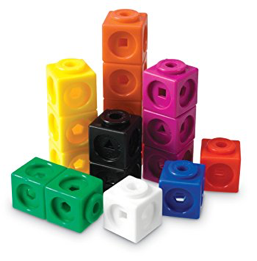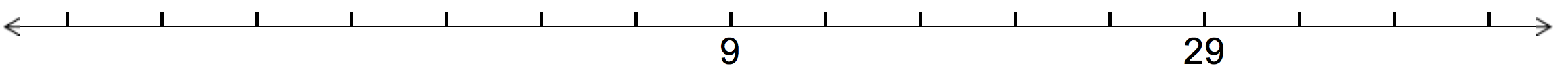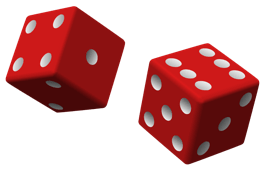# Problems to Ponder (March edition)

Welcome to this month’s edition of Problems to Ponder! Have an interesting solution? Send it to thevariable@smts.ca for publication in a future issue of The Variable!

Snap It! 

In this activity, students make different combinations for a given number.Materials
10 or more snap cubes per student

Each student makes a train of connecting cubes of a specified number. On the signal “Snap!”, the students break their trains into two parts and hold one hand behind their back.

In partners, or in a circle, students show one another their remaining cubes. The other students work out the number of cubes hiding behind their back.

Number Lines in Disguise 

This number line is missing some numbers.What number would be where the dot is? How do you know?Where would 0 be on the line?

Damult Dice 

In this game, students roll dice and try to reach a target number by adding and multiplying the numbers rolled.Materials

• 3 dice
• Paper and pencils, or white board and markers for recording work

Each player takes turns rolling the three dice.

On their turn, a player rolls two of the dice and adds the two numbers. Then, they roll the third die and multiply the sum by the number rolled. This player’s score for their turn is the sum of this product plus their previous score.

The first player to reach 200 (or 500, etc.) wins. Prior to the start of the game, players can decide whether the first person to exceed the target number wins, or whether the target number must be reached exactly. In the latter case, a player who cannot make a product that will not exceed the target number must skip their turn.

Adaptations: Play with dice numbered from 1 to 3 and make the target number 100. Give partners a hundreds chart and two tokens to keep track of their score

Triangles, Circles, Squares 

a) The large triangle below is equilateral. The red triangle is constructed using the midpoints of two sides, and a vertex, of the equilateral triangle.What fraction of the whole triangle is the red triangle?

b) Four identical semicircles with radius 2 are constructed in the red square below. What is the area of the square?Squares Squared 

I have four squares. One of them is painted red, one white, one blue, and one yellow, but otherwise they are indistinguishable. I wish to assemble them into one large square. How many ways can I assemble the square?Now assume that the squares are colored on both sides so that the assembled square can be turned over. In how many ways can I assemble the square?

Extend: Suppose that I now have eight cubes. Two of them are painted red, two white, two blue, and two yellow, but otherwise they are indistinguishable. I wish to assemble them into one large cube with each colour appearing on each face. In how many different ways can I assemble the cube?

Fred and Francine 

Fred and Francine are on a run from A to B. Fred runs half the way and walks the other half. Francine runs for half the time and walks for the other half. They both run and walk at the same speeds.Who finishes first?

Extend: Frank joins them and teaches them to jog. Fred now runs one-third of the way, jogs one-third of the way and walks the rest, while Francine jogs for one third of the time, runs for one third of the time, and walks the rest.

Who finishes first? Has Frank helped them to finish sooner or later than previously?

Sources